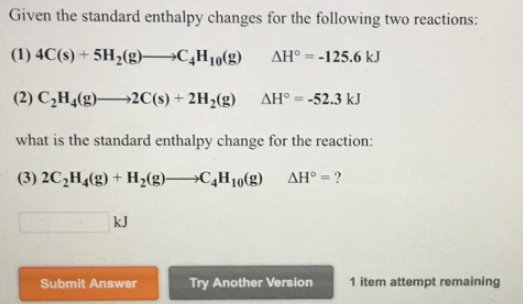# Problem: Given the standard enthalpy changes for the following two reactions: 4C (s) + 5H2 (g) → C4H10 (g)      ΔH° = -125.6 kJ C2H4 (g) → 2C (s) + 2H2 (g)       ΔH° = -52.3 kJ what is the standard enthalpy change for the reaction: 2C2H4 (g) + H2 (g) → C4H10 (g)  ΔH° = ?

###### FREE Expert Solution
82% (95 ratings)###### Problem Details

Given the standard enthalpy changes for the following two reactions:

4C (s) + 5H2 (g) → C4H10 (g)      ΔH° = -125.6 kJ

C2H4 (g) → 2C (s) + 2H2 (g)       ΔH° = -52.3 kJ

what is the standard enthalpy change for the reaction:

2C2H4 (g) + H2 (g) → C4H10 (g)  ΔH° = ?Frequently Asked Questions

What scientific concept do you need to know in order to solve this problem?

Our tutors have indicated that to solve this problem you will need to apply the Hess's Law concept. You can view video lessons to learn Hess's Law. Or if you need more Hess's Law practice, you can also practice Hess's Law practice problems.

What professor is this problem relevant for?

Based on our data, we think this problem is relevant for Professor Holland's class at SDSU.